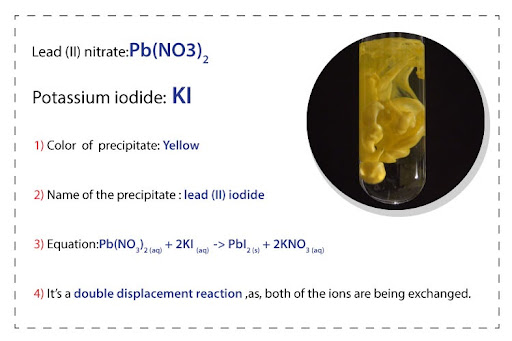# When the solutions of lead (II) nitrate and potassium iodide are mixed:What is the name and color of the precipitate formed?When the solutions of lead II nitrate and potassium class 11 chemistry CBSE

When the solutions of lead (II) nitrate and potassium iodide are mixed:
What is the name and color of the precipitate formed?
Whenever a question like this is asked, the first thing to do is write down the equation for the reaction. Let’s start with the reactants. We know that lead (II) nitrate and potassium iodide are the reactants. The formula for lead (II) nitrate contains the lead (II) cation and the nitrate anion:
Pb2+ and  NO3-1
These will combine to give the overall lead (II) nitrate formula accordingly, as we know that 2 nitrate ions combine with one lead ion, according to the charges: Pb(NO3)2
Hint: the oxidation state of lead is given in the word formula - lead (II)!
The formula for potassium iodide contains the potassium cation and the iodide anion:
K+1  and  I-1
These will combine to give the overall potassium iodide formula accordingly: KI
Next, we start the equation for this reaction -
Pb(NO3)2 + KI -> ?
The potassium cation reacts with the nitrate anion and the lead (II) cation reacts with the iodide anion in a double displacement reaction. This means that the products of the reaction will be:
Pb2+ + I-1  -> PbI2
K+ + NO3-1 -> KNO3
We can write these products in our reaction equation:
Pb(NO3)2 + KI -> PbI2 + KNO3
Next, balance the equation:
Pb(NO3)2 + 2KI -> PbI2 + 2KNO3
Finally, add the state symbols. According to the Salt Solubility Table, we know all Group 1 salts and nitrates are soluble in water, therefore they will have the following state symbols:
Pb(NO3)2 (aq) + 2KI(aq)  -> PbI2 + 2KNO3(aq)
We also know all iodides are soluble, except Ag+, Hg22+ and Pb2+, therefore PbI2 is an insoluble salt.
Pb(NO3)2 (aq) + 2KI(aq)  -> PbI2(s) + 2KNO3(aq)
Thus, the name of the precipitate formed is lead (II) iodide, which has a yellow appearance.